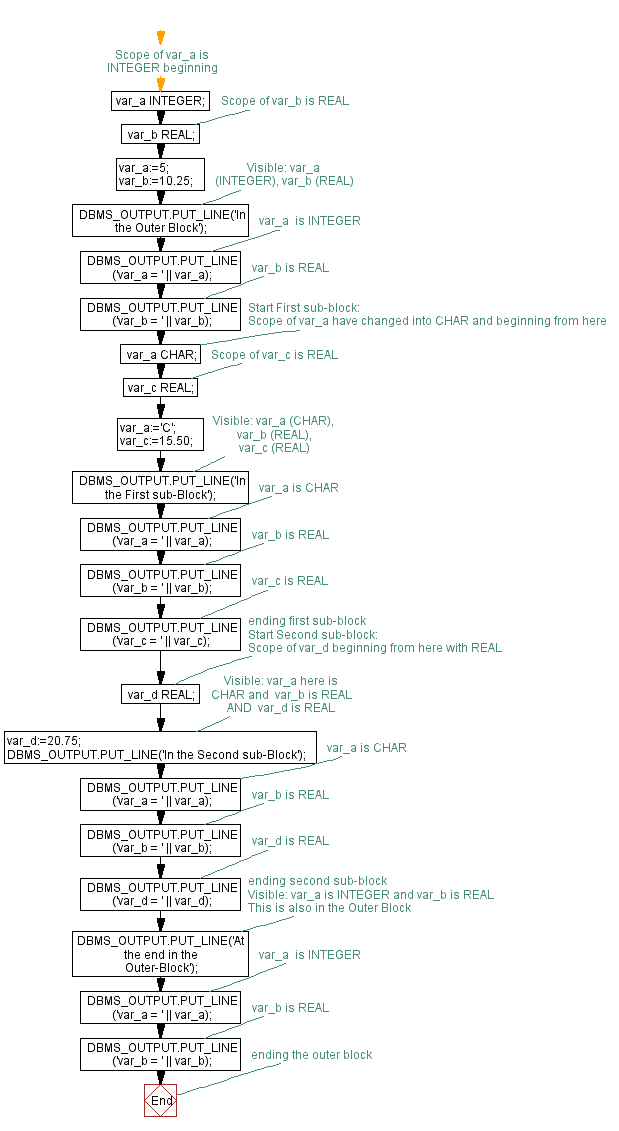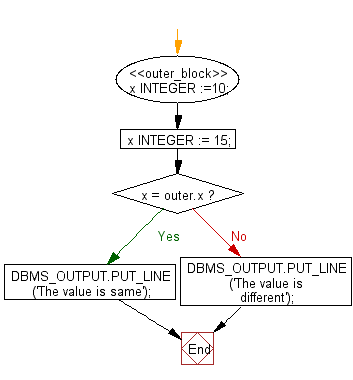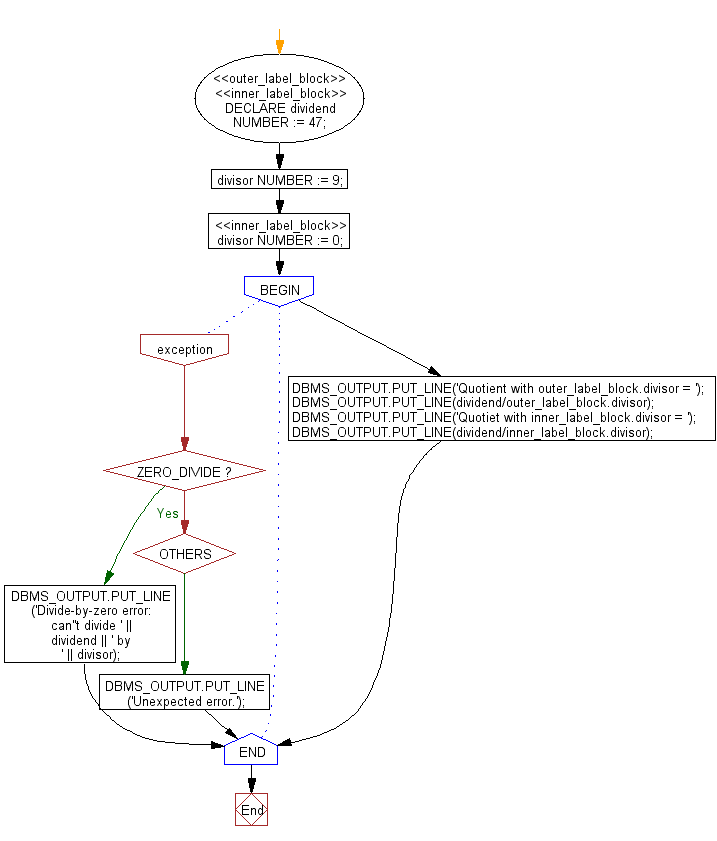﻿ PL/SQL Fundamentals Exercises: Scope and Visibility of Local and Global Identifiers - w3resource# PL/SQL Fundamentals Exercises: Scope and Visibility of Local and Global Identifiers

## PL/SQL Fundamentals: Exercise-8 with Solution

Write PL/SQL blocks to show the scope and visibility of local and global identifiers.

The following PL/SQL block shows how the scope and visibility of several identifiers can be defined. In the first sub-block the global identifier var_a have declared again. To reference the global variable var_a, the first sub-block would have to qualify it with the name and data type of the outer block. So, the first sub-block cannot reference the global variable var_a; it can reference only its local variable var_a. Therefore the first sub-block cannot reference var_d, and the second sub-block cannot reference var_c.

PL/SQL Code:

``````DECLARE
var_a INTEGER;  -- Scope of var_a is INTEGER beginning
var_b REAL;    -- Scope of var_b is REAL
BEGIN
var_a:=5;
var_b:=10.25;
-- Visible: var_a (INTEGER), var_b (REAL)
DBMS_OUTPUT.PUT_LINE('In the Outer Block');
DBMS_OUTPUT.PUT_LINE('var_a = ' || var_a); -- var_a  is INTEGER
DBMS_OUTPUT.PUT_LINE('var_b = ' || var_b); -- var_b is REAL

-- Start First sub-block:
DECLARE
var_a CHAR;  -- Scope of var_a have changed into CHAR and beginning from here
var_c REAL;       -- Scope of var_c is REAL
BEGIN
var_a:='C';
var_c:=15.50;

-- Visible: var_a (CHAR), var_b (REAL), var_c (REAL)
DBMS_OUTPUT.PUT_LINE('In the First sub-Block');
DBMS_OUTPUT.PUT_LINE('var_a = ' || var_a); -- var_a is CHAR
DBMS_OUTPUT.PUT_LINE('var_b = ' || var_b); -- var_b is REAL
DBMS_OUTPUT.PUT_LINE('var_c = ' || var_c); -- var_c is REAL
NULL;
END;          --  ending first sub-block

-- Start Second sub-block:
DECLARE
var_d REAL;     -- Scope of var_d beginning from here with REAL
BEGIN
-- Visible: var_a here is CHAR and  var_b is REAL AND  var_d is REAL
var_d:=20.75;
DBMS_OUTPUT.PUT_LINE('In the Second sub-Block');
DBMS_OUTPUT.PUT_LINE('var_a = ' || var_a); -- var_a is CHAR
DBMS_OUTPUT.PUT_LINE('var_b = ' || var_b); -- var_b is REAL
DBMS_OUTPUT.PUT_LINE('var_d = ' || var_d); -- var_d is REAL
NULL;
END;          -- ending second sub-block

-- Visible: var_a is INTEGER and var_b is REAL
-- This is also in the Outer Block
DBMS_OUTPUT.PUT_LINE('At the end in the  Outer-Block');
DBMS_OUTPUT.PUT_LINE('var_a = ' || var_a); -- var_a  is INTEGER
DBMS_OUTPUT.PUT_LINE('var_b = ' || var_b); -- var_b is REAL
END;            --   ending the outer block
/
```
```

Sample Output:

```In the Outer Block
var_a = 5
var_b = 10.25
In the First sub-Block
var_a = C
var_b = 10.25
var_c = 15.5
In the Second sub-Block
var_a = 5
var_b = 10.25
var_d = 20.75
At the end in the  Outer-Block
var_a = 5
var_b = 10.25

Statement processed.

0.01 seconds
```

Flowchart:Write a PL/SQL block to show how to declared a global identifier again for qualifying in block label.

In the following example outer block have defined with the name outer_block as a label. Therefore, after the sub-block the global variable x have declared again, it can reference that global variable by qualifying its name with the block label. The sub-block can also reference its local variable x, by its simple name.

PL/SQL Code:

``````<<outer_block>>  -- outer label
DECLARE
x INTEGER :=10;
BEGIN
DECLARE
x INTEGER := 15;
BEGIN
IF x = outer.x THEN
DBMS_OUTPUT.PUT_LINE ('The value is same');
ELSE
DBMS_OUTPUT.PUT_LINE ('The value is different');
END IF;
END;
END;
/
```
```

Sample Output:

```The value is different

Statement processed.

0.14 seconds

```

Flowchart:Write a PL/SQL block to show how to declared multiple and duplicate label block.

In the following example two labels have been defined for the outer block, outer_label_block and inner_label_block. The inner_label_block appears again in the inner block. In the inner block, inner_label_block.divisor refers to the local variable divisor, not to the global variable divisor, which results in the error ZERO_DIVIDE.

PL/SQL Code:

``````<<outer_label_block>>
<<inner_label_block>>
DECLARE
dividend   NUMBER := 47;
divisor NUMBER := 9;
BEGIN
<<inner_label_block>>
DECLARE
divisor NUMBER := 0;
BEGIN
DBMS_OUTPUT.PUT_LINE('Quotient with outer_label_block.divisor = ');
DBMS_OUTPUT.PUT_LINE(dividend/outer_label_block.divisor);

DBMS_OUTPUT.PUT_LINE('Quotiet with inner_label_block.divisor = ');
DBMS_OUTPUT.PUT_LINE(dividend/inner_label_block.divisor);

EXCEPTION
WHEN ZERO_DIVIDE THEN
DBMS_OUTPUT.PUT_LINE('Divide-by-zero error: can''t divide '
|| dividend || ' by ' || divisor);
WHEN OTHERS THEN
DBMS_OUTPUT.PUT_LINE('Unexpected error.');
END inner_label_block;
END outer_label_block;
/
```
```

Flowchart:Sample Output:

```Quotient with outer_label_block.divisor =
5.22222222222222222222222222222222222222
Quotiet with inner_label_block.divisor =
Divide-by-zero error: can't divide 47 by 0

Statement processed.

0.13 seconds
```

Improve this sample solution and post your code through Disqus

What is the difficulty level of this exercise?

﻿